# Category: Plotting

## Matplotlib Histogram

Matplotlib can be used to create histograms. A histogram shows the frequency on the vertical axis and the horizontal axis is another dimension. Usually it has bins, where every bin has a minimum and maximum value. Each bin also has a frequency between x and infinite.

Related course

Matplotlib histogram example
Below we show the most minimal Matplotlib histogram:

Output: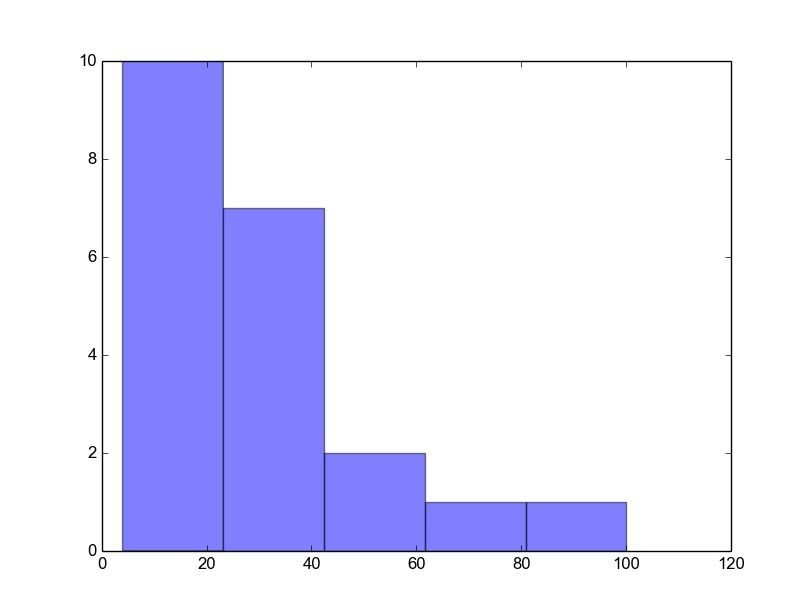Python histogram

A complete matplotlib python histogram
Many things can be added to a histogram such as a fit line, labels and so on. The code below creates a more advanced histogram.

Output: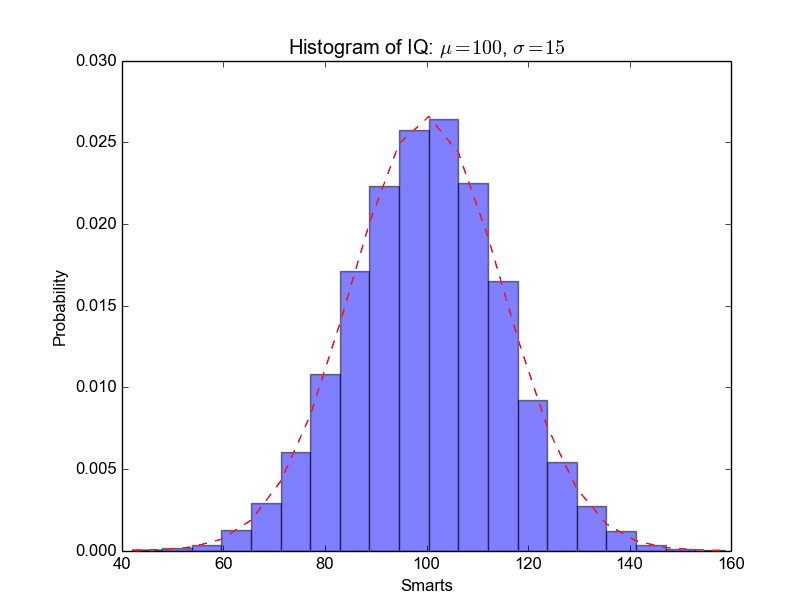python_histogram

## Matplotlib Line chart

A line chart can be created using the Matplotlib plot() function. While we can just plot a line, we are not limited to that. We can explicitly define the grid, the x and y axis scale and labels, title and display options.

Related course:

Line chart example
The example below will create a line chart.

Output: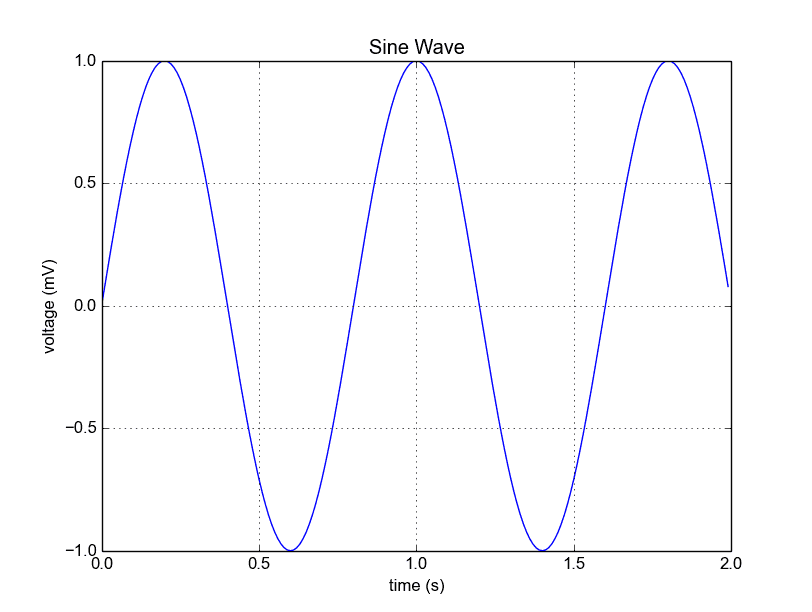Python Matplotlib Line Chart

The lines:

simply define the data to be plotted.

plots the chart.  The other statements are very straightforward: statements xlabel() sets the x-axis text, ylabel() sets the y-axis text, title() sets the chart title and grid(True) simply turns on the grid.

If you want to save the plot to the disk, call the statement:

Plot a custom Line Chart

If you want to plot using an array (list), you can execute this script:

The statement:

defines start from 0, plot 20 items (length of our array) with steps of 1.

Output: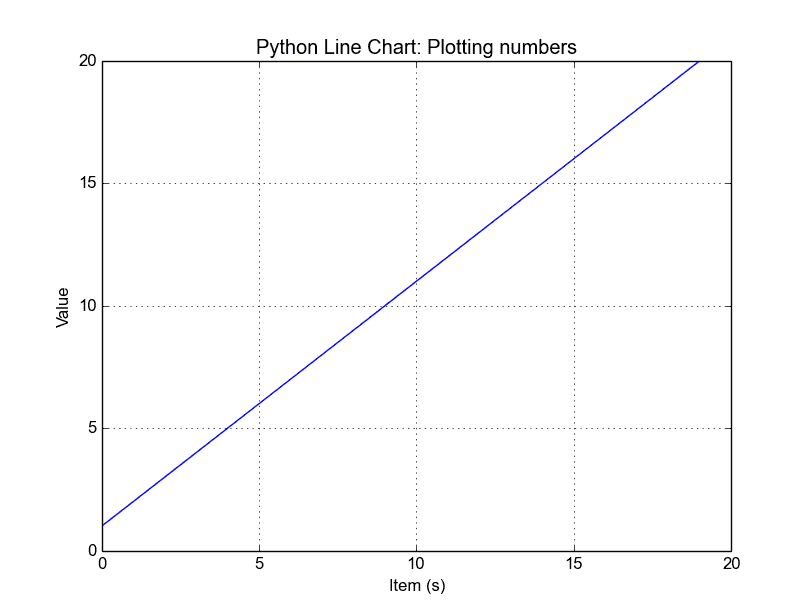Python Line Chart from List

Multiple plots

If you want to plot multiple lines in one chart, simply call the plot() function multiple times. An example:

Output: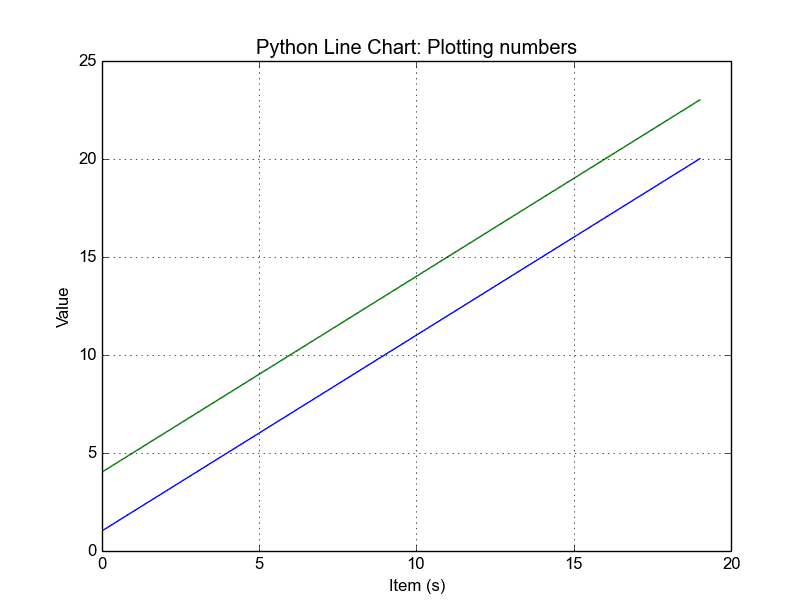python line chart multiple

In case you want to plot them in different views in the same window you can use this:

Output: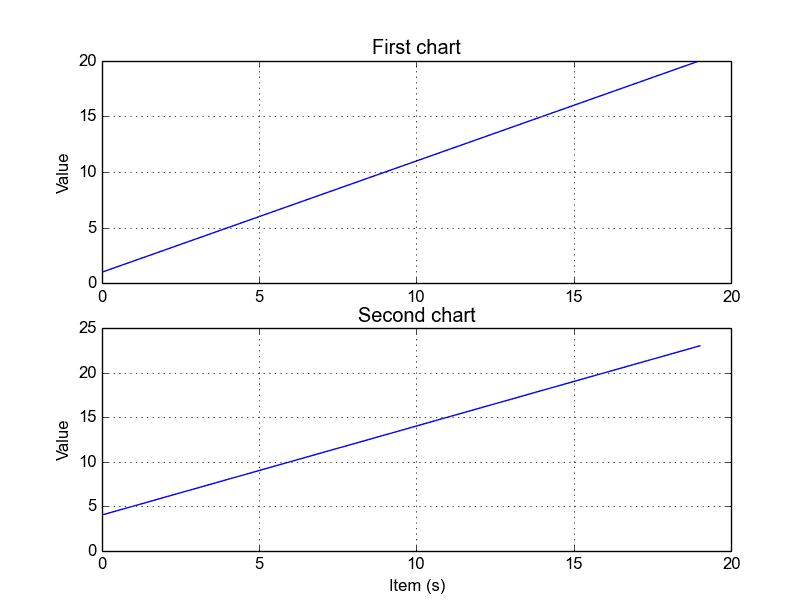Python subplots

The plt.subplot() statement is key here. The subplot() command specifies numrows, numcols and fignum.

Styling the plot
If you want thick lines or set the color, use:

## Matplotlib Bar chart

Matplotlib may be used to create bar charts. You might like the Matplotlib gallery.

Matplotlib is a python library for visualizing data. You can use it to create bar charts in python. Installation of matplot is on pypi, so just use pip: pip install matplotlib

The course below is all about data visualization:

Related course:
Data Visualization with Matplotlib and Python

## Bar chart code

A bar chart shows values as vertical bars, where the position of each bar indicates the value it represents. matplot aims to make it as easy as possible to turn data into Bar Charts.

A bar chart in matplotlib made from python code. The code below creates a bar chart:

Output: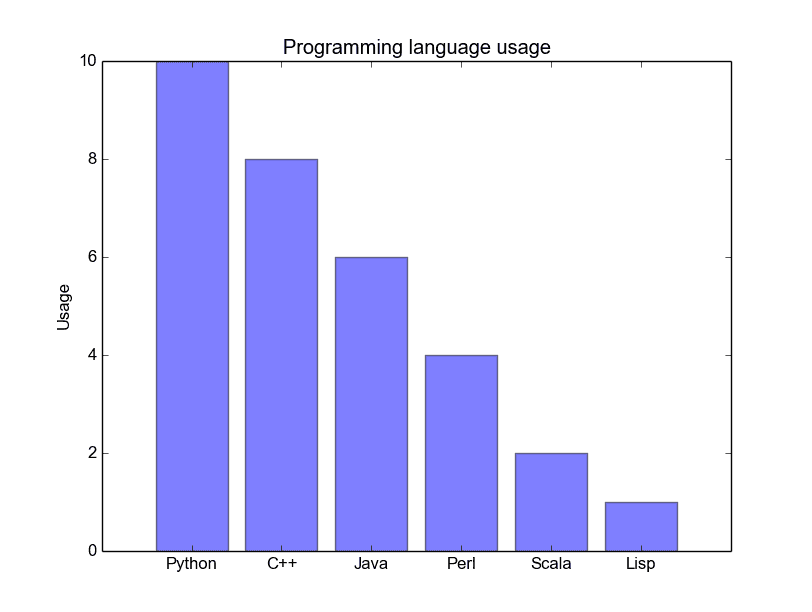Python Bar Chart

Matplotlib charts can be horizontal, to create a horizontal bar chart:

Output: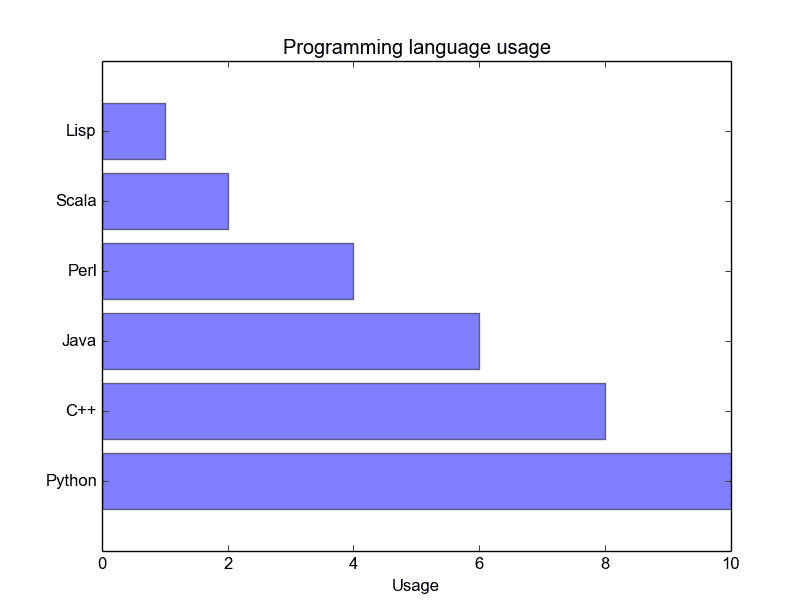Bar chart horizontal

## Bar chart comparison

You can compare two data series using this Matplotlib code:

Output: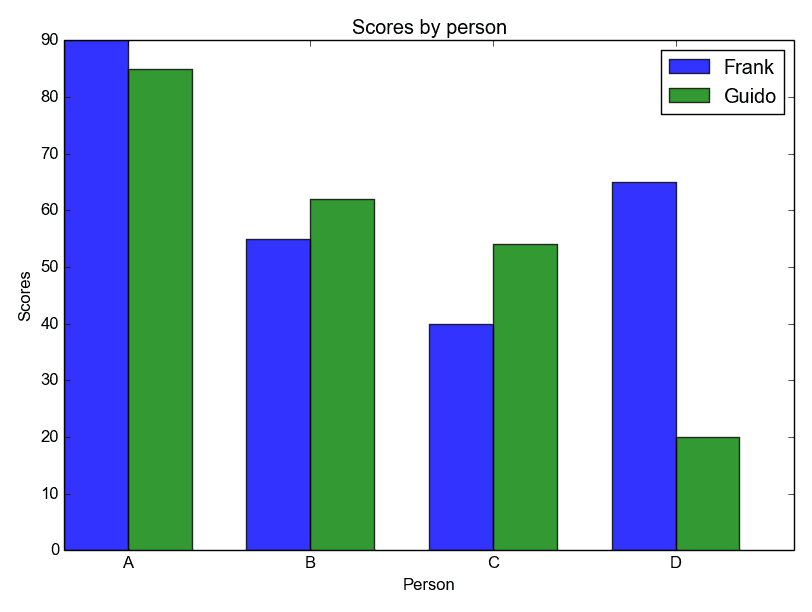Python Bar Chart comparison

## Stacked bar chart

The example below creates a stacked bar chart with Matplotlib. Stacked bar plots show diffrent groups together.

Output:## Matplotlib Pie chart

Matplotlib supports pie charts using the pie() function. You might like the Matplotlib gallery.

The matplotlib module can be used to create all kinds of plots and charts with Python. A pie chart is one of the charts it can create, but it is one of the many.

Related course: Data Visualization with Matplotlib and Python

## Matplotlib pie chart

First import plt from the matplotlib module with the line import matplotlib.pyplot as plt
Then you can use the method plt.pie() to create a plot.

The code below creates a pie chart:

The above code has the following output: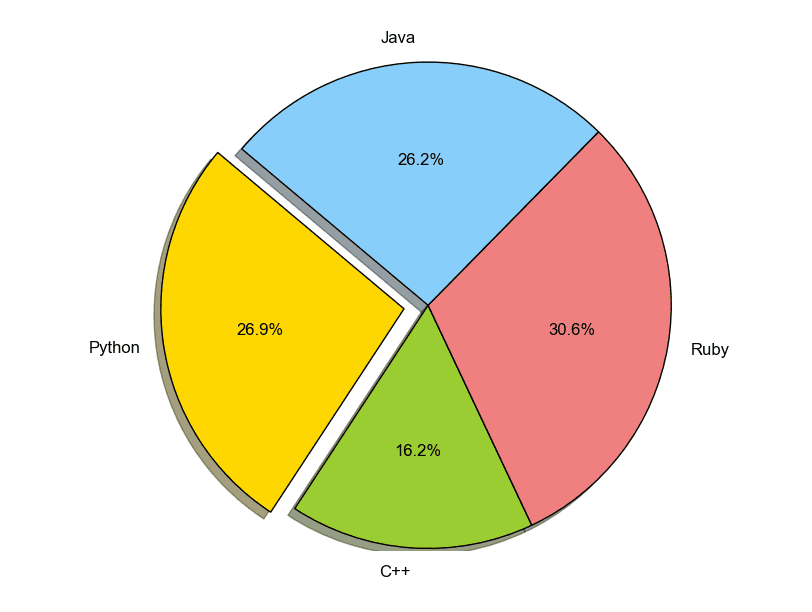pie chart python

You can define it’s sizes, which parts should explode (distance from center), which labels it should have and which colors it should have.

## Matplotlib pie chart legend

To add a legend use the plt.legend() function. This adds a legend on top of the plot.

It outputs this plot: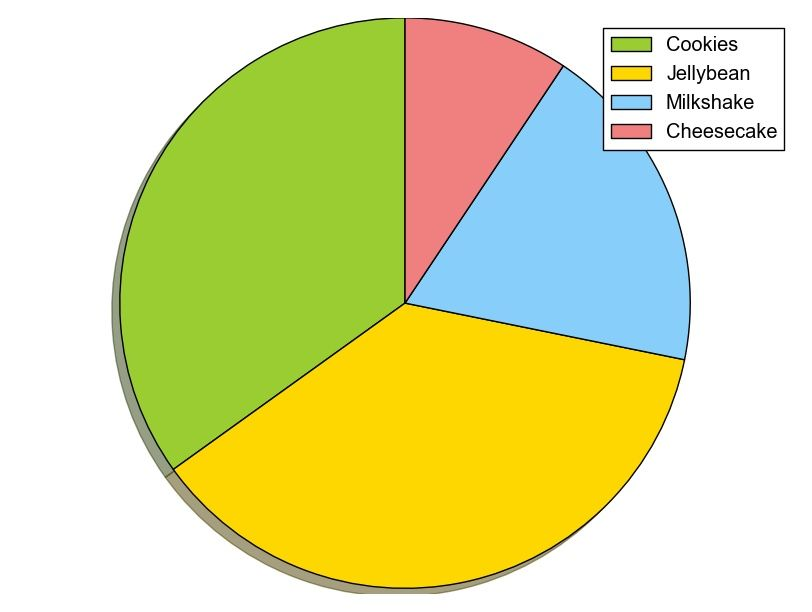python pie chart

While making the plot, don’t forget to call the method .show().

## Matplotlib legend

Matplotlib has native support for legends. Legends can be placed in various positions: A legend can be placed inside or outside the chart and the position can be moved.

The legend() method adds the legend to the plot. In this article we will show you some examples of legends using matplotlib.

Related course

Matplotlib legend inside
To place the legend inside, simply call legend():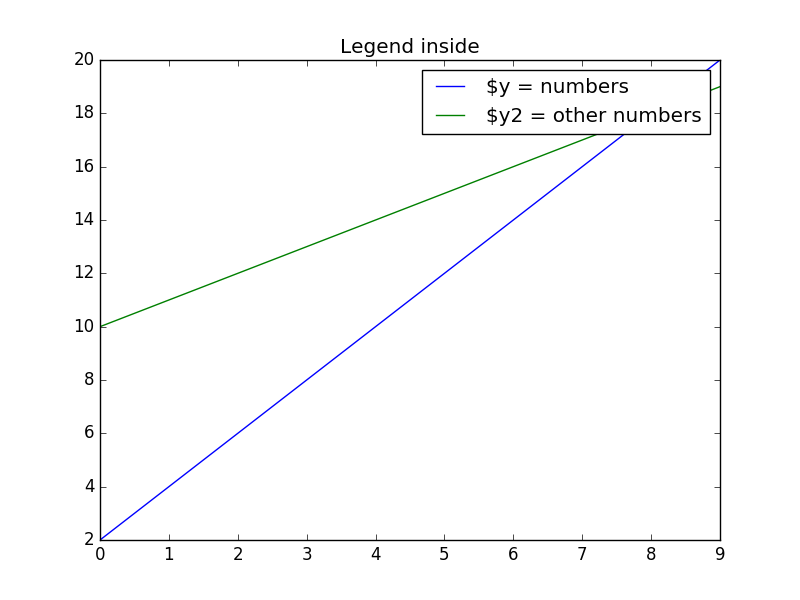Matplotlib legend inside

Matplotlib legend on bottom
To place the legend on the bottom, change the legend() call to:

Take into account that we set the number of columns two ncol=2 and set a shadow.

The complete code would be: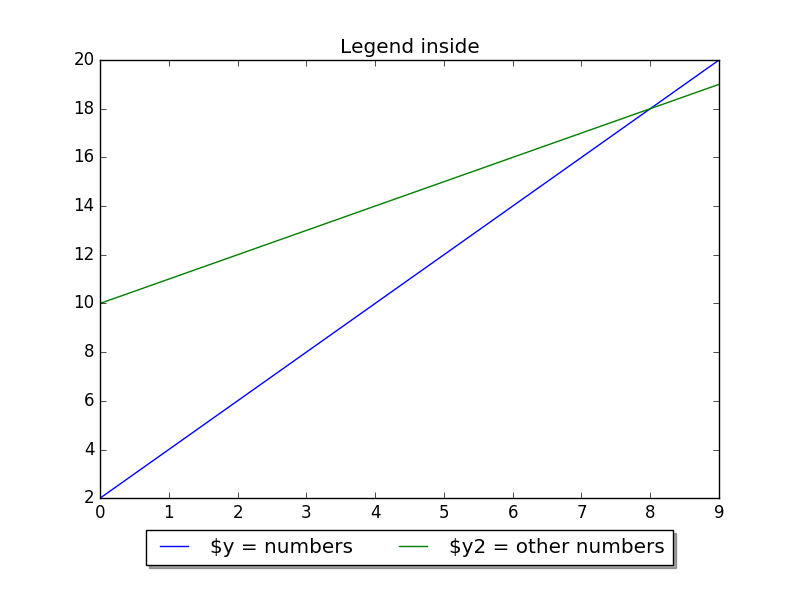Legend placed on bottom

Matplotlib legend on top
To put the legend on top, change the bbox_to_anchor values:

Code: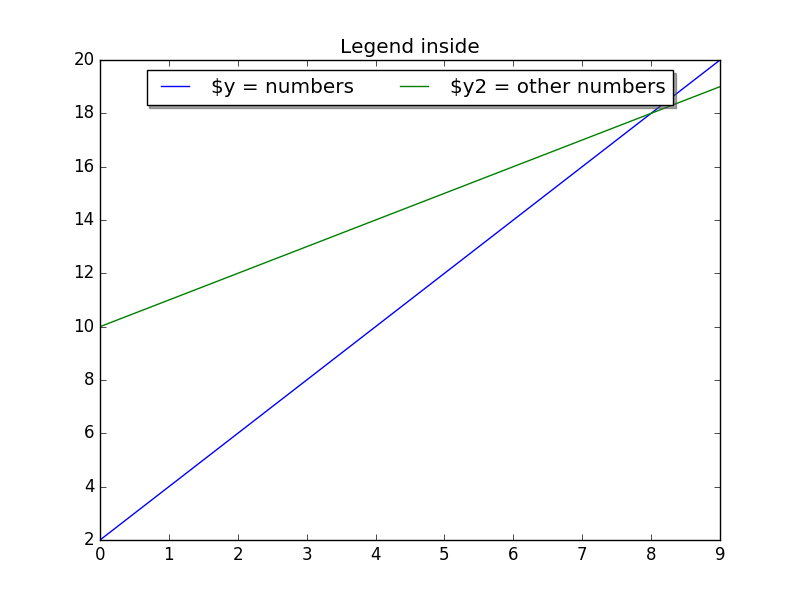Legend on top

Legend outside right
We can put the legend ouside by resizing the box and puting the legend relative to that:

Code: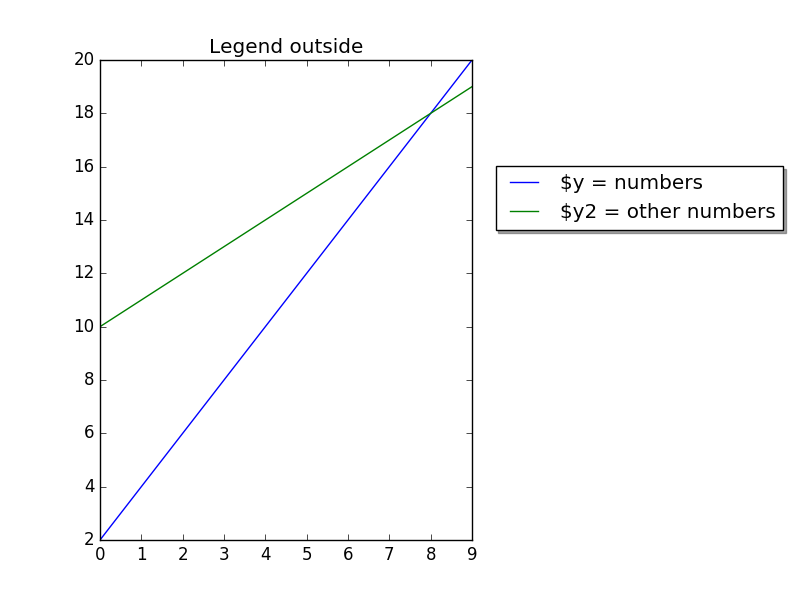Matplotlib legend outside

## Matplotlib save figure to image file

If you want to save matplotlib figures as individual files, you can do this with the savefig function. If you want to save figures in a single file, use the saveas function instead.

Matplotlib is a python plotting library which produces publication quality figures in a variety of hardcopy formats and interactive environments across platforms.

Related course
The course below is all about data visualization:
Data Visualization with Matplotlib and Python

## Save figure

For those who didn’t know, matplotlib savefig creates a file of the current figure as a PNG (with transparency), and saves it to your filesystem.

So Matplotlib can save plots directly to a file using savefig(). In this article we won’t cover the installation of matplotlib, if you want to install it see the installation faq.

Savefig is useful when you need to save a figure for viewing outside of matplotlib, such as in another program, or when you want to use a vector graphics program (Inkscape, Illustrator, etc.) to modify it.

It’s also useful if you want to save a copy of a figure in the same directory as your matplotlib script.

The method can be used like this:

It can make an image from the figure. It decides on the image format based on the extension. For example to save a jpg image named figure1. jpg. The figure image must have an extension of jpg, png, or pdf.

## The savefig method

The savefig() method is part of the matplotlib.pyplot module. This saves the contents of your figure to an image file.

It must have the output file as the first argument. You can add the full path, relative path or no path. If you don’t define a path, it will save the image in the current working directory.

The most basic program you can do is just 5 lines of code:

This works for larger plots too:

To change the format, simply change the extension like so:

You can open your file with any external image program, because it’s just a regular image. If you use Linux, you can use the command below:

or open it in an image or pdf viewer if you saved it as pdf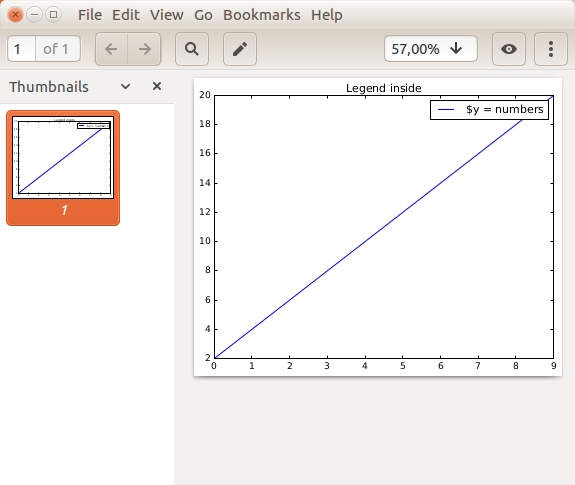A plot saved to a pdf

A number of new savefig options have been added to matplotlib. Backwards compatibility is maintained.
The options are:

The output file name extension and format is controlled by the extension and format parameters; the above are defaults.

Useful parameters are:

• filename the output file to save, if no path is included it will save it in the same directory as your program

• transparent if you a transparent background set it to True

• bbox_inches change the size of the white space around the image, in most cases tight is ideal

## Save as PDF file

To save your matplotlib chart as a pdf, just change the file extension to .pdf

The file will be saved in your working directory.

## Save as SVG file

SVG is another vector-based graphics format, which lets you zoom in without losing quality. Not every program can open the svg image files.

To save as an SVG file, just change the extension to SVG

## Save as JPEG file

If you save as jpeg file, you can include the quality parameter. This lets you save some disk space, but at the cost of image quality.

In general I recommend going with vector graphics formats like svg or pdf, because the quality is higher. If you don’t care about quality and just want to email the image or show it on a website, you could use png or jpeg.

## Matplotlib update plot

Updating a matplotlib plot is straightforward. Create the data, the plot and update in a loop.
Setting interactive mode on is essential: plt.ion(). This controls if the figure is redrawn every draw() command. If it is False (the default), then the figure does not update itself.

Related course:

## Update plot example

Copy the code below to test an interactive plot.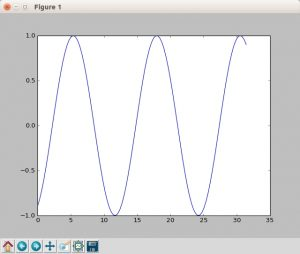Capture of a frame of the program above

Explanation
We create the data to plot using:

Turn on interacive mode using:

Configure the plot (the ‘b-‘ indicates a blue line):

And finally update in a loop:

## Plot time with matplotlib

Matplotlib supports plots with time on the horizontal (x) axis. The data values will be put on the vertical (y) axis. In this article we’ll demonstrate that using a few examples.

It is required to use the Python datetime module, a standard module.

Related course

Plot time
You can plot time using a timestamp: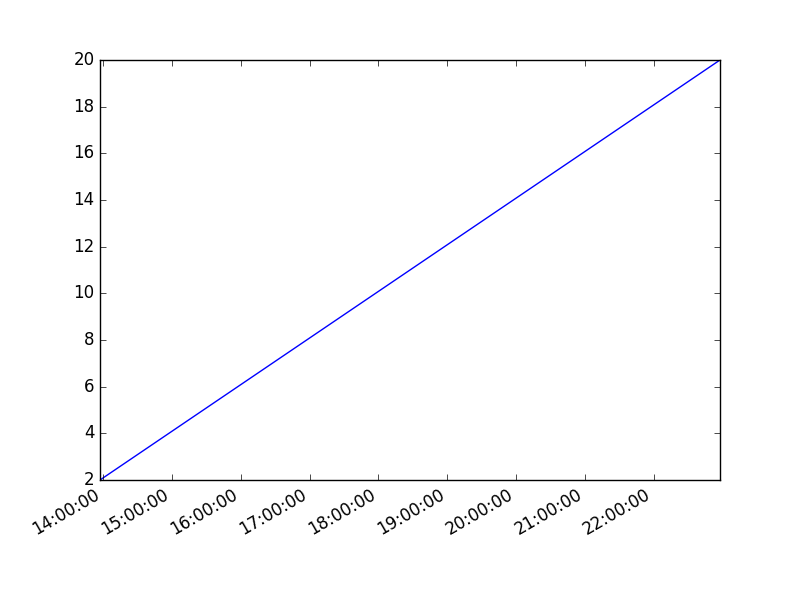If you want to change the interval use one of the lines below:

Time plot from specific hour/minute

To start from a specific date, create a new timestamp using datetime.datetime(year, month, day, hour, minute).
Full example:

## Generate heatmap in Matplotlib

A heatmap can be created using Matplotlib and numpy.

Related courses
If you want to learn more on data visualization, this course is good:

## Heatmap example

The histogram2d function can be used to generate a heatmap.

We create some random data arrays (x,y) to use in the program. We set bins to 64, the resulting heatmap will be 64x64. If you want another size change the number of bins.

Result: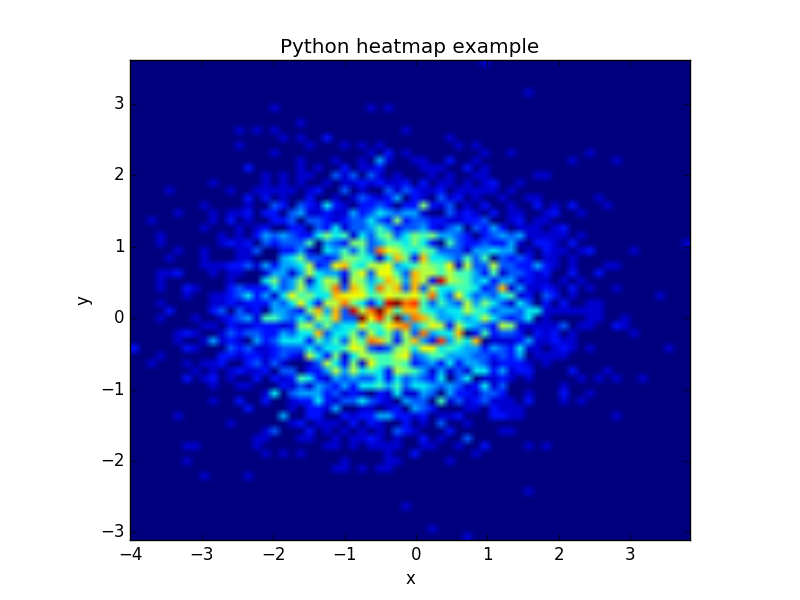Matplotlib heatmap

The datapoints in this example are totally random and generated using np.random.randn()

## Matplotlib scatterplot

Matplot has a built-in function to create scatterplots called scatter(). A scatter plot is a type of plot that shows the data as a collection of points. The position of a point depends on its two-dimensional value, where each value is a position on either the horizontal or vertical dimension.

Related course

Scatterplot example
Example: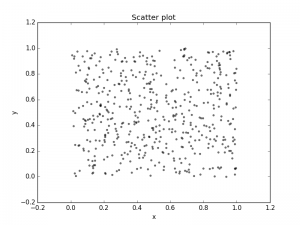Scatter plot created with Matplotlib

Scatter plot with groups
Data can be classified in several groups. The code below demonstrates that:

Related course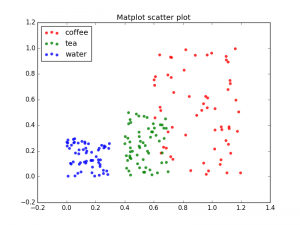Scatter plot with classes

## 3d scatterplot

Matplotlib can create 3d plots. Making a 3D scatterplot is very similar to creating a 2d, only some minor differences. On some occasions, a 3d scatter plot may be a better data visualization than a 2d plot. To create 3d plots, we need to import axes3d.

Related course:

Introduction
It is required to import axes3d:

Give the data a z-axis and set the figure to 3d projection:

## 3d scatterplot

Complete 3d scatterplot example below:

The plot is created using several steps:

• vector creation (g1,g2,g3)

• list creation (groups)

• plotting

The final plot is shown with plt.show()

## Matplotlib Subplot

The Matplotlib subplot() function can be called to plot two or more plots in one figure. Matplotlib supports all kind of subplots including 2x1 vertical, 2x1 horizontal or a 2x2 grid.

Related courses

• Data Visualization with Matplotlib and Python

• Horizontal subplot
Use the code below to create a horizontal subplot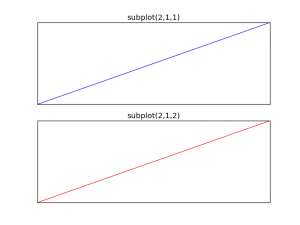matplotlib subplot

Vertical subplot
By changing the subplot parameters we can create a vertical plot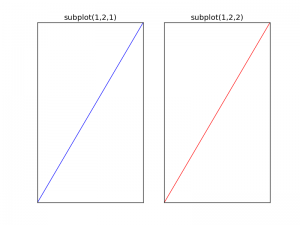matplotlib subplot vertical

Subplot grid
To create a 2x2 grid of plots, you can use this code:

## Matplot Matrix Correlation

A correlation diagram can be created using Matplotlib. Matplotlib is the most used plotting library for Python. It can be included in all the graphical toolkits that are available for Python.

Related courses

• Data Visualization with Matplotlib and Python

• Matrix definition
To start we define a 2x2 matrix and a list called groups. The matrix is defined inside the brackets (double [[ and ]] if written on a single line).

Visual:

## Matrix correlation

The code below generates a Matrix correlation diagram using Matplotlib.

Initially we define the matrix (m) and the list (groups).
We set the length to be equal to the length of the groups. On the x axis and y axis we set the group names.